# Moment of inertia for particle – problems and solutions

1. Two balls connected by a rod, as shown in the figure below. Ignore rod’s mass. Mass of ball P is 600 gram and mass of ball Q is 400 gram. What is the moment of inertia of the system about AB?

Known :

The axis of rotation is AB.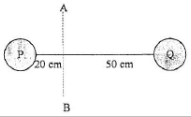mp = 600 gram = 0.6 kg, mq = 400 gram = 0.4 kg

rp = 20 cm = 0.2 m, rq = 50 cm = 0.5 m

Wanted : The moment of inertia of the system

Solution :

I = mp rp2 + mq rq2

I = (0.6 kg)(0.2 m)2 + (0.4 kg)(0.5 m)2

I = (0.6 kg)(0.04 m2) + (0.4 kg)(0.25 m2)

I = 0.024 kg m2 + 0.1 kg m2

I = 0.124 kg m2

[irp]

2. The Rod of AB with mass of 2-kg rotated about point A, the moment of inertia of the rod is 8 kg m2. If rotated about point O (AO = OB),what is the moment of inertia of the rod.

Known :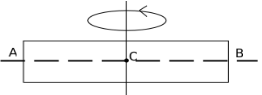Mass of rod AB (m) = 2 kg

If rotated about point A so that the radius of rotation (r) = length of AB = r then the moment of inertia (I) = 8 kg m2

Wanted: If rotated about point O so that the radius of rotation (r) = length of AO = length of OB = 1/2 r then what is the moment of inertia of the rod.

Solution :

I = m r2

8 kg m2 = (2 kg) r2

8 m2 = (2) r2

r2 = 8 m2 / 2

r2 = 4 m2

r = 2 meters

If rotated about point O so ½ r = 1 meter, then the moment of inertia :

I = m r2 = (2 kg)(1 m)2 = (2 kg)(1 m2) = 2 kg m2

[irp]

3. Two balls connected by a rod as shown in figure below. Ignore rod’s mass. What is the moment of inertia of the system.

Known :

Mass of ball A (mA) = 200 gram = 0.2 kg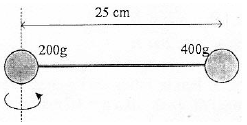Mass of ball B (mB) = 400 gram = 0.4 kg

Distance between ball A and the axis of rotation (rA) = 0

Distance between ball B and the axis of rotation (rB) = 25 cm = 0.25 m

Wanted : Moment of inertia of the system

Solution :

The moment of inertia of ball A :

IA = (mA)(rA2) = (0.2)(0)2 = 0

The moment of inertia of ball B :

IB = (mB)(rB2) = (0.4)(0.25)2 = (0.4)(0.0625) = 0.025 kg m2

The moment of inertia of system :

I = IA + IB = 0 + 0.025 = 0.025 kg m2 = 25 x 10-3 kg m2

[irp]

4. Four particles with different mass, shown in figure below. Determine the moment of inertia of the system about the horizontal line P.

Solution

The axis of rotation is the horizontal line P.

Known :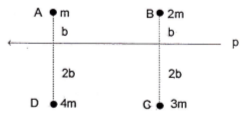Mass of particle A (mA) = m

Mass of particle B (mB) = 2m

Mass of particle C (mC) = 3m

Pass of particle D (mD) = 4m

Distance between particle A and the axis of rotation (rA) = b

Distance between particle B and the axis of rotation (rB) = b

Distance between particle C and the axis of rotation (rC) = 2b

Distance between particle D and the axis of rotation (rD) = 2b

Wanted : The moment of inertia of the system about the horizontal line P

Solution :

I = mA rA2 + mB rB2 + mC rC2 + mD rD2

I = (m)(b)2 + (2m)(b)2 + (3m)(2b)2 + (4m)(2b)2

I = mb2 + 2 mb2 + (3m)(4b2) + (4m)(4b2)

I = mb2 + 2 mb2 + 12 mb2 + 16 mb2

I = 31 mb2

[irp]

5. Four particles connected by a rod. Ignore rod’s mass. Determine the moment of inertia about the axis of rotation through particle m1 and m2, as shown in figure below.

Known

Mass of particle 1 (m1) = 1/4 kg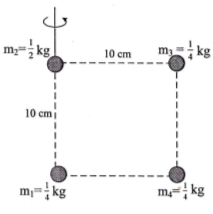Mass of particle 2 (m2) = 1/2 kg

Mass of particle 3 (m3) = 1/4 kg

Mass of particle 4 (m4) = 1/4 kg

Distance between particle 1 and the axis of rotation (r1) = 0

Distance between particle 2 and the axis of rotation (r2) = 0

Distance between particle 3 and the axis of rotation (r3) = 10 cm = 10/100 m = 1/10 m

Distance between particle 4 and the axis of rotation (r4) = 10 cm = 10/100 m = 1/10 m

Wanted : The moment of inertia

Solution :

I = m1 r12 + m2 r22 + m3 r32 + m4 r42

I = (1/4)(0)2 + (1/2)(0)2 + (1/4)(1/10)2 + (1/4)(1/10)2

I = 0 + 0 + (1/4)(1/100) + (1/4)(1/100)

I = 1/400 + 1/400

I = 2/400

I = 1/200 kg.m2